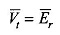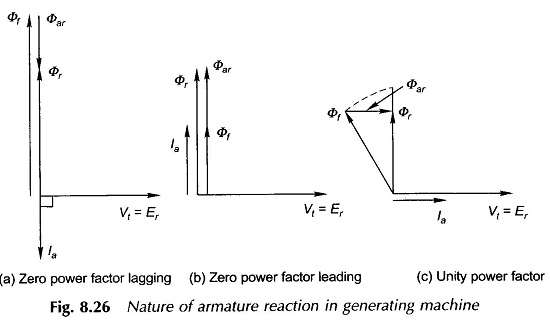## Effect of Armature Reaction:

The Effect of Armature Reaction is dependent on the power factor at which the machine is operating and upon the operating mode generating/motoring. For simplicity of explanation, it will be assumed here that the armature resistance and leakage reactance are negligible so thatFigure 8.26 shows the phasor diagrams, with component fluxes (i.e. the magnetic circuit is assumed linear) indicated therein for a generating machine for

1. Zero power factor lagging (Fig. 8.26(a))
2. Zero power factor leading (Fig. 8.26(b)) and
3. For unity power factor (Fig. 8.26(c)).

#### The following observations are immediately made from these phasor diagrams.

1. Armature reaction is demagnetizing (Φar opposes Φf) when a generating machine supplies zero power factor lagging current.
2. Armature reaction is magnetizing (Φar aids Φf) when a generating machine supplies zero power factor leading current.
3. Armature reaction is mostly cross-magnetizing (i.e. at 90° to Φf) though it has a small demagnetizing component (see dotted phasors in Fig. 8.26(c), when a generating machine supplies unity power factor current.From the above discussion the following more general conclusions regarding a synchronous machine in generating mode can be drawn:

• When the machine supplies a lagging power factor current, the armature reaction has both demagnetizing and cross-magnetizing components.
• When the machine supplies leading power factor current, the armature reaction has both magnetizing and cross-magnetizing components.

Since in a motoring machine the armature reaction mmf and flux are in phase opposition to the armature current, the Effect of Armature Reaction is just the reverse of what is stated above for the generating machine.

#### The corresponding conclusions for the motoring machine are stated below:

• When the machine draws a lagging power factor current, the armature reac­tion has both magnetizing and cross-magnetizing components.
• When the machine draws leading power factor current, the Effect of Armature Reaction has both demagnetizing and cross-magnetizing components.